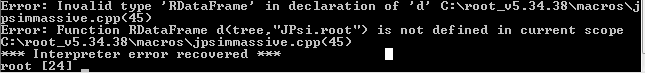Hello people! How to read the branch momentum.fCoordinates.fX in tree? SetBranchAddress gives nulls.
Peace of code:
Double_t m_x = { 0 };

for (int ev = 0; ev <5; ev++) {

``````	tree->GetEntry(ev);

for (int j = 0; j < 4; j++) {

cout << m_x[j] << "	";
v2[j].SetXYZT(m_x[j], m_y[j], m_z[j], m_t[j]);

}
cout << endl;
``````

}

You need to have equivalent types for it to work. You will probably either have to use `tree->SetBranchAddress(“momentum.fCoordinates”, &m_x);` or set it for each component individually. In any case, have you looked at RDataFrame? It’s probably easier to use it to process your data.Does it mean that in ROOT 5.34 version not include RDataFrame ?

That’s right, only ROOT 6.14 and above have `RDataFrame`. In ROOT 6.10 and 6.12 it was called `TDataFrame` and was part of the `ROOT::Experimental` namespace.

This topic was automatically closed 14 days after the last reply. New replies are no longer allowed.